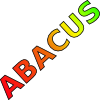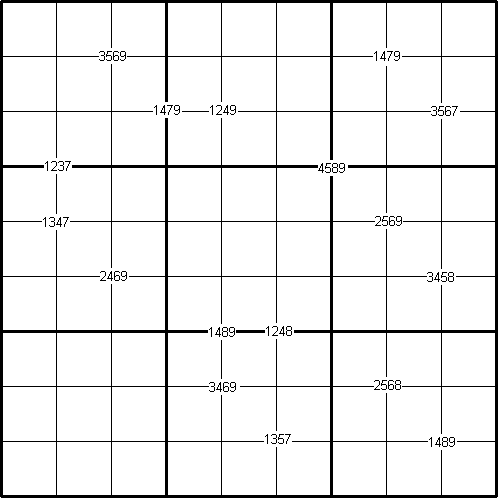#### You may also like### Consecutive Numbers

An investigation involving adding and subtracting sets of consecutive numbers. Lots to find out, lots to explore.### Tea Cups

Place the 16 different combinations of cup/saucer in this 4 by 4 arrangement so that no row or column contains more than one cup or saucer of the same colour.### Counting on Letters

The letters of the word ABACUS have been arranged in the shape of a triangle. How many different ways can you find to read the word ABACUS from this triangular pattern?

##### Age 11 to 16Challenge Level

By Henry Kwok#### Rules of Quadruple Clue Sudoku

This is a variation of sudoku on a "standard" $9 \times 9$ grid which contains a set of special clue-numbers. These are small numbers provided by sets of $4$ small digits.
Each set of $4$ small digits in the intersection of two grid lines stands for the numbers in the four cells of the grid adjacent to this set.

The remaining rules are as in a "standard" sudoku, and the object of the puzzle is to fill in the whole $9 \times 9$ grid with numbers $1$ through $9$ so that each row, each column, and each of the nine $3\times 3$ boxes must contain all the nine different numbers $1$ through $9$.

For example, in the puzzle, taking the two sets of adjacent cells with small digits {$1479$} and {$3567$}, we find that they overlap at the cell with the number $7$. The rest of the puzzle is solved in the same way through logical deduction using the usual sudoku techniques and by determining the numbers in the cells in one set that overlap the cells in other sets.